# lcheng

•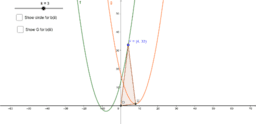### DSE2019Q19

Activity

lcheng

•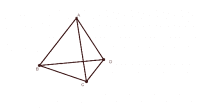### Regular Tetrahedron

Activity

lcheng

•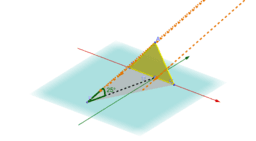### Sunrays with different angles

Activity

lcheng

•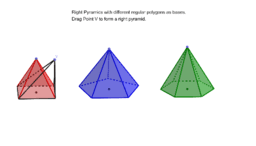### Right Pyramids

Activity

lcheng

•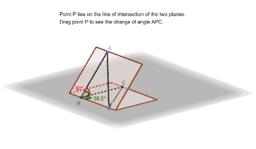### Angle between Two Planes (Basic)

Activity

lcheng

•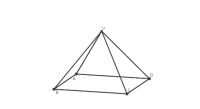### Pyramid with square base (angle between planes)

Activity

lcheng

•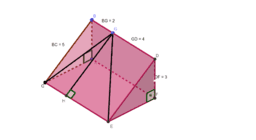### Inclined plane (Angle between two planes)

Activity

lcheng

•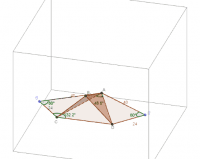### DSE15 Q19 Example

Activity

lcheng

•### Cube (Angle between a line and a plane)

Activity

lcheng

•### Enlargement and Reduction along x-axis

Activity

lcheng

•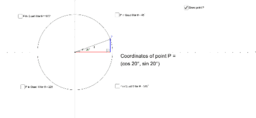### Trigonometric ratios in four Quadrants

Activity

lcheng

•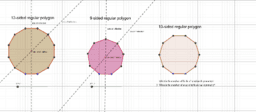### Rotation Symmetry and Reflectional Symmetry of Regular Polygon

Activity

lcheng

•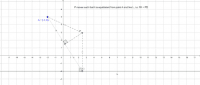### Equidistant from a fixed point and a fixed line

Activity

lcheng

•### Equidistant from two parallel lines

Activity

lcheng

•### Property of Equilateral Triangle

Activity

lcheng

•### Sides Opposite Equal Angles

Activity

lcheng

•### Angle of Rotation

Activity

lcheng

•### Maintains two perpendicular lines

Activity

lcheng

•### Equidistant from two fixed lines

Activity

lcheng

•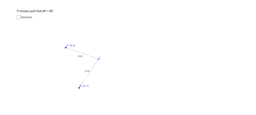### Equal distance from two fixed points

Activity

lcheng

•### a fixed distance from a line

Activity

lcheng

•### a fixed distance from a line segment

Activity

lcheng

•### A fixed distance from a fixed point

Activity

lcheng

•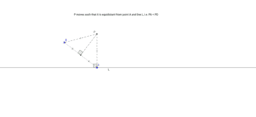Activity

lcheng